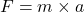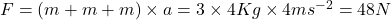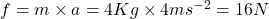## Three identical 4.0-kg cubes are placed on a horizontal frictionless surface in contact with one another. The cubes are lined up from left t

Question

Three identical 4.0-kg cubes are placed on a horizontal frictionless surface in contact with one another. The cubes are lined up from left to right and a force is applied to the left side of the left cube causing all three cubes to accelerate to the right at 4.0 m/s2 . What is the magnitude of the force exerted on the right cube by the middle cube in this case

in progress 0
4 months 2021-09-04T08:10:57+00:00 1 Answers 0 views 0

## Answers ( )

The amount of force exerted by the middle cube on the right cube will be 16 N.

Explanation:

Newton’s second law of motion says that the force acting on some object is directly proportional to the rate of change of momentum of the object and the change occurs in the same direction in which the force is applied. If due to the application of a force (F) an object of mass (m) gains an acceleration (a), mathematically we can writeAs according to the problem all the cubes are attached to each other, so the amount of force applied can be written asThis force will be uniformly distributed among the three cubes to generate the acceleration. So if ‘f‘ is the force exerted by the middle cube on the right one then,